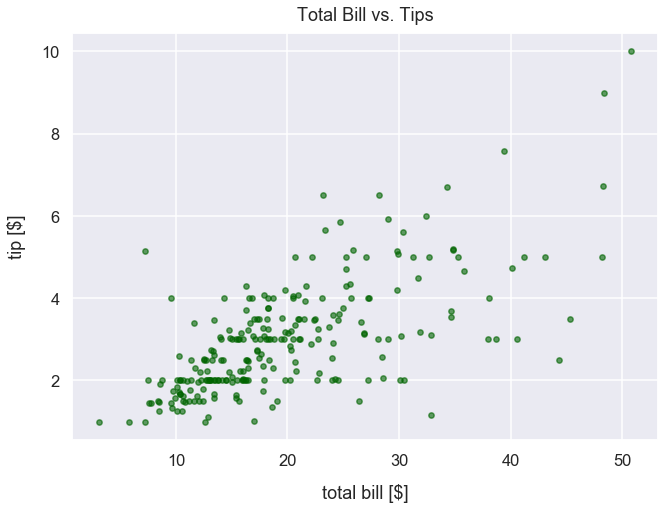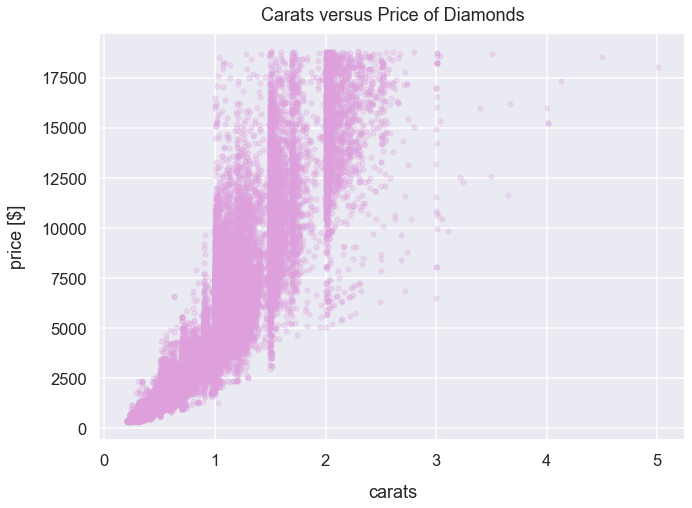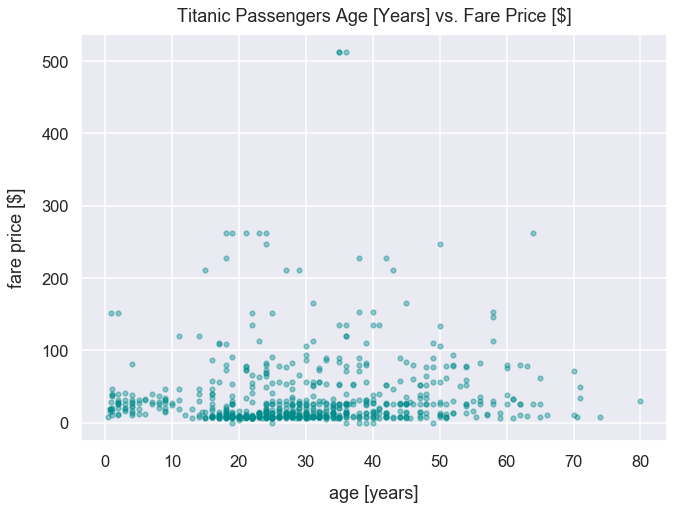Math Descriptive Statistics Article

# Correlation

In statistics, the Pearson correlation coefficient is a measure of linear correlation between two variables of data.

In business context, the term correlation is used to to describe a relationship between two or more things. This is an alternative use case than what we'll cover in this article which is just the pearson correlation coefficient.

This tutorial will illustrate the pearson correlation through scatter plots and analysis of the exact correlation coefficient values.

### Explanation of Coefficient Values¶

If the correlation coefficient is positive, as values from one variable increase, so does the other.

If the correlation coefficient is negative, as values from one variable decrease, the values from other variable increase.

The table below provides some examples of Pearson's correlation coefficient values and explanations.

Pearson's correlation coefficient Interpretation
0 There's no correlation between the two variables
-0.25 There's a small negative correlation between the two variables
-0.75 There's a large negative correlation between the two variables
-1.0 There's a perfect negative correlation between the two variables
0.25 There's a small positive correlation between the two variables
0.75 There's a large positive correlation between the two variables
1.0 There's a perfect positive correlation between the two variables

### Assumption to Prove Correlation¶

Generally, for both variables compared, we want them to be roughly normally distributed. The reason for this is that it's unfair to interpret a correlation pattern if we're just looking at extremes of values.

For example, let's say we want to understand the relationship between income and net worth. We'd initially assume there's a positive linear relationship between the two variables: as income increases, net worth increases. However, hypothetically, let's say our dataset just had net income values around 0-20,00 U.S. dollars and 150,000-500,000 U.S. dollars. In this instance, our income values wouldn't represent a normal distribution. We're missing lots of income values between 20,000 and 150,000 U.S. dollars - which I think is a fairly common range for U.S. workers.

We could still compare income and net worth and we'd likely see the people in that small income range have low net worth values while the people in that much higher net income range have larger net worth values. Yet, it's unfair to interpret correlation between these two variables since we're neglecting a huge portion of people with a certain income and it's biased to connect a relationship between just the extremes of income data.

Let's move onto some visual examples now to explain correlation.

### Import Modules¶

In :
import pandas as pd
import seaborn as sns
import scipy.stats as stats
import matplotlib.pyplot as plt
%matplotlib inline


Visualization styling code

In :
sns.set(rc={'figure.figsize':(10.5, 7.5)})
sns.set_context('talk')


### Example 1: Tips Dataset - Total Bill and Tip¶

#### Get the Data¶

Let's get the tips dataset from the seaborn library and assign it to the DataFrame df_tips.

In :
df_tips = sns.load_dataset('tips')


Each row represents a unique meal at a restaurant for a party of people; the dataset contains the following fields:

column name column description
total_bill financial amount of meal in U.S. dollars
tip financial amount of the meal's tip in U.S. dollars
sex gender of server
smoker boolean to represent if server smokes or not
day day of week
time meal name (Lunch or Dinner)
size count of people eating meal

Preview the first 5 rows of df_tips.

In :
df_tips.head()

Out:
total_bill tip sex smoker day time size
0 16.99 1.01 Female No Sun Dinner 2
1 10.34 1.66 Male No Sun Dinner 3
2 21.01 3.50 Male No Sun Dinner 3
3 23.68 3.31 Male No Sun Dinner 2
4 24.59 3.61 Female No Sun Dinner 4

#### Plot the Relationship Between Total Bill and Tip Amounts¶

The plot below has total bill values on the x-axis and tips on the y-axis.

In :
df_tips.plot.scatter(x='total_bill', y='tip', s=28, alpha=0.6, c='darkgreen')
plt.xlabel("total bill [$]", labelpad=15) plt.ylabel("tip [$]", labelpad=15)
plt.title("Total Bill vs. Tips", y=1.015);There seems to be a large positive correlation between the two variables. As total bill sizes increase, tip values increase too. This is what we'd expect since tips given at restaurants are typically 20% of total bill size, so there's mutual dependence between these two variables.

#### Evaluate the Pearson's Correlation Coefficient Between Tips and Bill Amounts¶

We can use the scipy packages's stats module and pearsonr() method to compute the Pearson correlation coefficient.

This method returns a list in which the first value is the correlation coefficient.

Our returned value is 0.68 which confirms our belief that there's a large positive correlation between the two variables.

In :
round(stats.pearsonr(df_tips['tip'], df_tips['total_bill']), 2)

Out:
0.68

We can also the use DataFrame method corr() from the pandas package to compute a pairwise correlation of columns.

There'll always be a perfect positive correlation, a coefficient of 1, for each variable with itself. The 0.68 value below is the same as the one from our calculation with scipy above.

In :
df_tips[['tip', 'total_bill']].corr()

Out:
tip total_bill
tip 1.000000 0.675734
total_bill 0.675734 1.000000

### Example 2: Diamonds Dataset - Carat and Price¶

#### Get Diamonds Dataset¶

Import diamonds dataset from Seaborn library and assign to DataFrame df_diamonds.

Each row of df_diamonds contains details about a specific diamond purchased. We'll just utilize the price and carat columns below.

In :
df_diamonds = sns.load_dataset('diamonds')


Preview the first few rows of df_diamonds.

In :
df_diamonds.head()

Out:
carat cut color clarity depth table price x y z
0 0.23 Ideal E SI2 61.5 55.0 326 3.95 3.98 2.43
1 0.21 Premium E SI1 59.8 61.0 326 3.89 3.84 2.31
2 0.23 Good E VS1 56.9 65.0 327 4.05 4.07 2.31
3 0.29 Premium I VS2 62.4 58.0 334 4.20 4.23 2.63
4 0.31 Good J SI2 63.3 58.0 335 4.34 4.35 2.75

#### Explanation of carats¶

carat is a unit of measurement in diamonds to characterize its mass. Typically, bigger/heavier diamonds are known to be more expensive.

Here's a helpful image from womengettingmaried.com.#### View Distribution of Carats versus Diamond Prices¶

The plot below has carats on the x-axis and price on the y-axis.

In :
df_diamonds.plot.scatter(x='carat', y='price', s=22, alpha=0.25, c='plum')
plt.ylabel("price [$]", labelpad=15) plt.title("Carats versus Price of Diamonds", y=1.015);There seems to be a large positive correlation between the two variables. As carats increases, price increases too. This is expected since we assume larger/heavier diamonds are priced higher than smaller ones. #### Evaluate the Pearson's Correlation Coefficient Between Tips and Bill Amounts¶ In : round(stats.pearsonr(df_diamonds['carat'], df_diamonds['price']), 2)  Out: 0.92 Our returned value is 0.92 means there's a very strong (nearly perfect) correlation between the two variables. ### Example 3: Titanic Dataset - Age and Fare Price¶ #### Get Titanic Dataset¶ Import titanic dataset from Seaborn library and assign to DataFrame df_titanic. Each row of df_titanic represents details of a passenger of the ship. We'll just utilize the age and fare columns below. The fare column represents the price a passenger paid to board the ship. In : df_titanic = sns.load_dataset('titanic')  Let's create a new DataFrame assigned to the variable df_titanic_non_nulls that filters out the rows in the age column that were nulls. In : df_titanic_non_nulls = df_titanic[df_titanic['age'].notnull()==True]  #### View Distribution of Age vs. Fare Prices¶ The plot below has age in years on the x-axis and price in U.S. dollars on the y-axis. In : df_titanic_non_nulls.plot.scatter(x='age', y='fare', s=22, alpha=0.4, c='darkcyan') plt.xlabel("age [years]", labelpad=15) plt.ylabel("fare price [$]", labelpad=15)
plt.title("Titanic Passengers Age [Years] vs. Fare Price [\$]", y=1.015);There seems to be no clear correlation between the two variables. The visualization just illustrates just a bunch of random dots.

#### Evaluate the Pearson's Correlation Coefficient Between Age and Fare Price¶

In :
round(stats.pearsonr(df_titanic_non_nulls['age'], df_titanic_non_nulls['fare']), 1)

Out:
0.1

Our returned value is 0.1 means there's essentially no correlation between the two variables. A change in the value of one variable has no expected change in the value of the other variable.

### Correlation Does Not Imply Causation¶

One of the most popular terms in the field of statistics is "correlation does not imply causation". A correlation means there's a linear relationship between two variables. A causation connects one process, the cause, with another process or state, the effect.

However, this does not mean a change in one variable causes a change in the value of the other variable.

For example, there are studies that have likely proved that increased exercise correlates with a decrease in a person's mass. This is considered to be a strong negative correlation between the two variables. This relationship has also likely been proven to be cause-and-effect in which once the activity of exercising takes place, this causes the decrease in a person's mass.

On the other hand, here's a different example mentioned in this Wikediia article: as ice cream sales increase, the rate of drowning deaths increases sharply. These two variables can be correlated. So as ice cream sales have increased over time, so have drowning deaths so there's a positive linear relationship between the two variables. Yet, it's incredibly unlikely there there's any causuality relationship between those two variables since they're so distinct from one another. Rather, people eat more ice cream in warm-weather months and also likely swim more in these months. If more people are swimming, there's likely a greater chance of drowning deaths. The fact is: ice cream consumption does not cause drowning. There's lots of other factors to consider in this relationship such as weather.